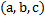# Find the distance of the-axis from the image of the pointin the plane## Question ID - 153285 :- Find the distance of the-axis from the image of the pointin the plane3537

 (1) If image of pointis the planeis, thenHence the image is (0, 1, 5) Obviously distance of image of the point from-axis is 1
 The position vectors of the four angular points of a tetrahedronare (0, 0, 0), (0, 0, 2), (0, 4, 0) and (6, 0, 0), respectively. A pointinside the tetrahedron is at the same distance ‘’ from the four plane faces of the tetrahedron. Find the value of